Case Based Questions (MCQ)

Chapter 3 Class 12 Matrices
Serial order wise

## Three schools SNT, SNP and TKM organised a mela  for collecting funds for helping the rehabilitation of  flood victims. They sold hand-made fans, mats and  plates from recycled material at a cost of ₹ 25, ₹ 100 and  ₹ 50 each. The number of articles sold are given below.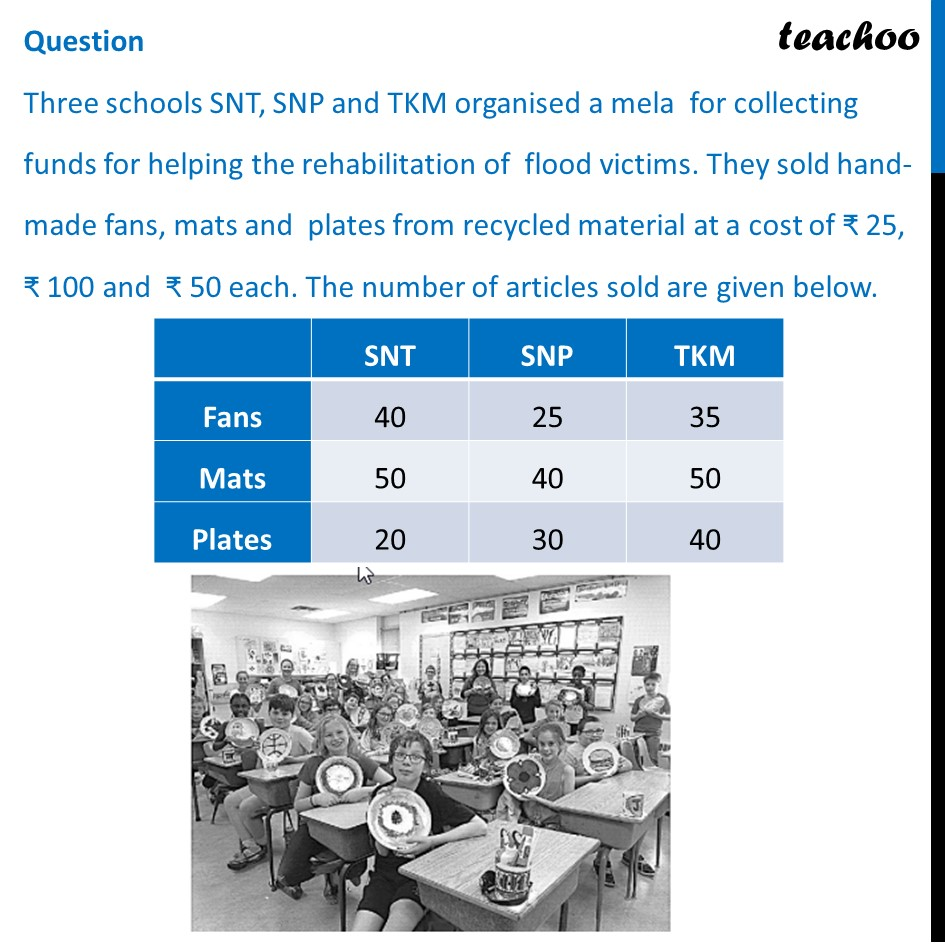## (d) Rs. 21000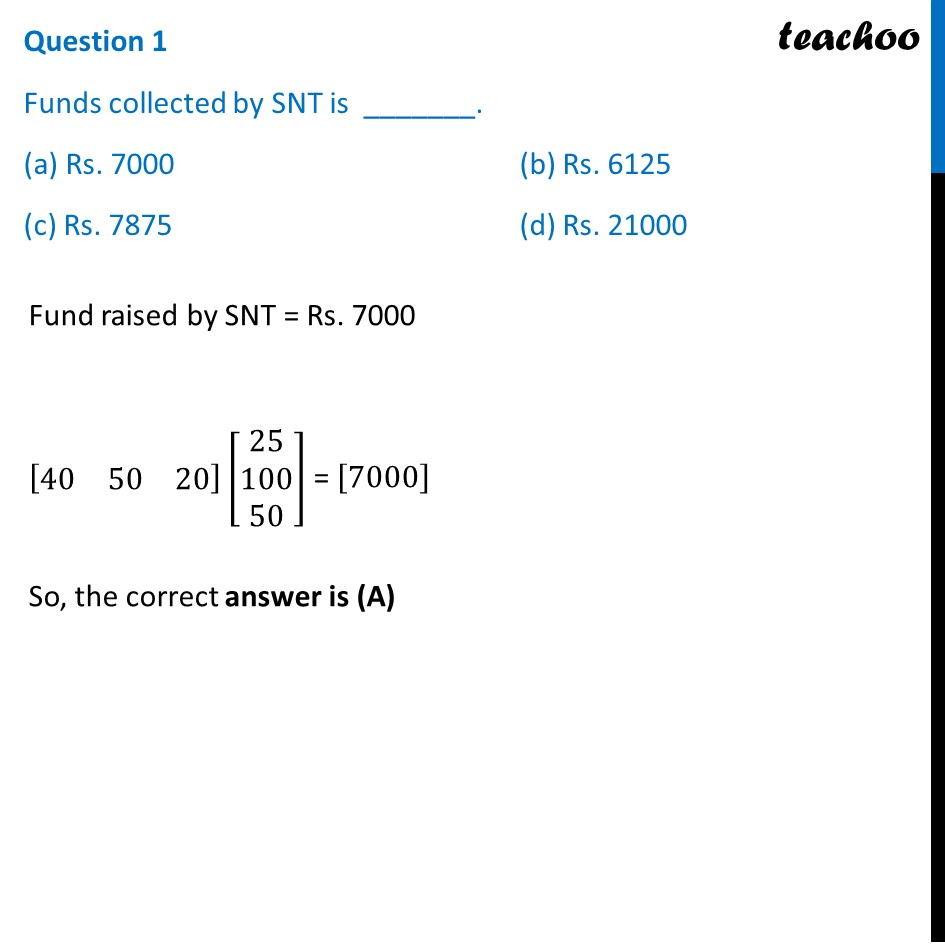## (d) Rs. 21000## (d) Rs. 21000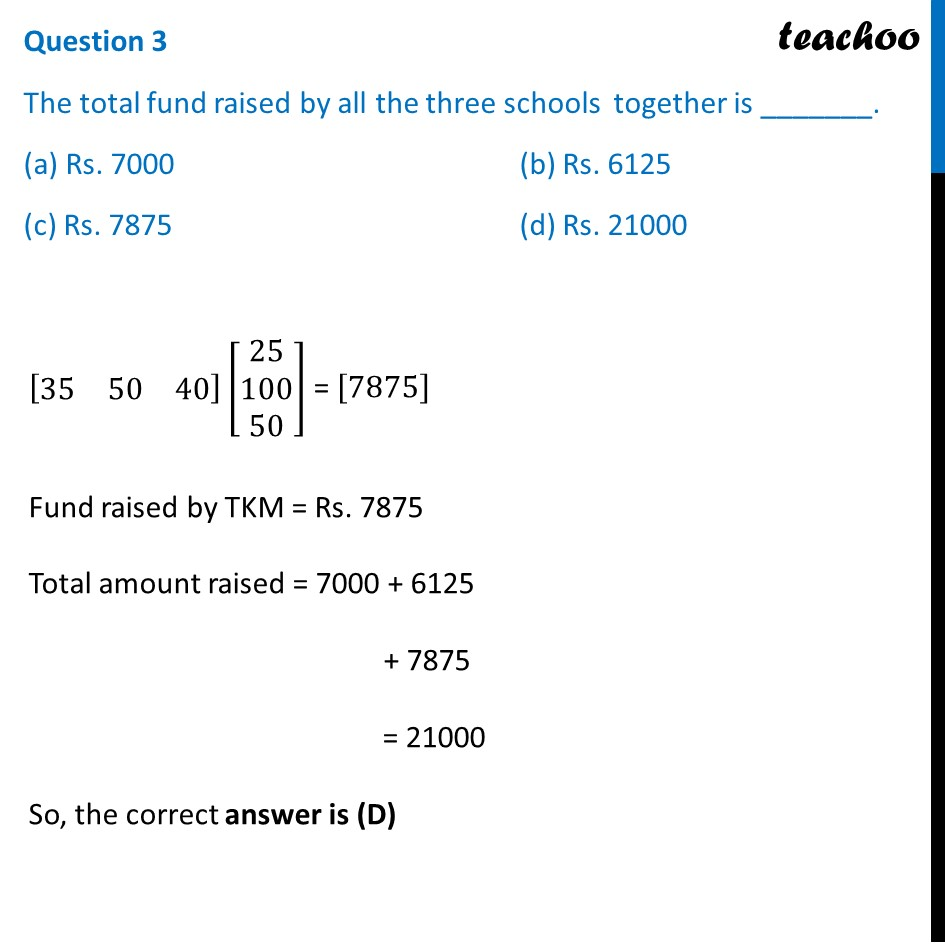## (d) Rs. 35000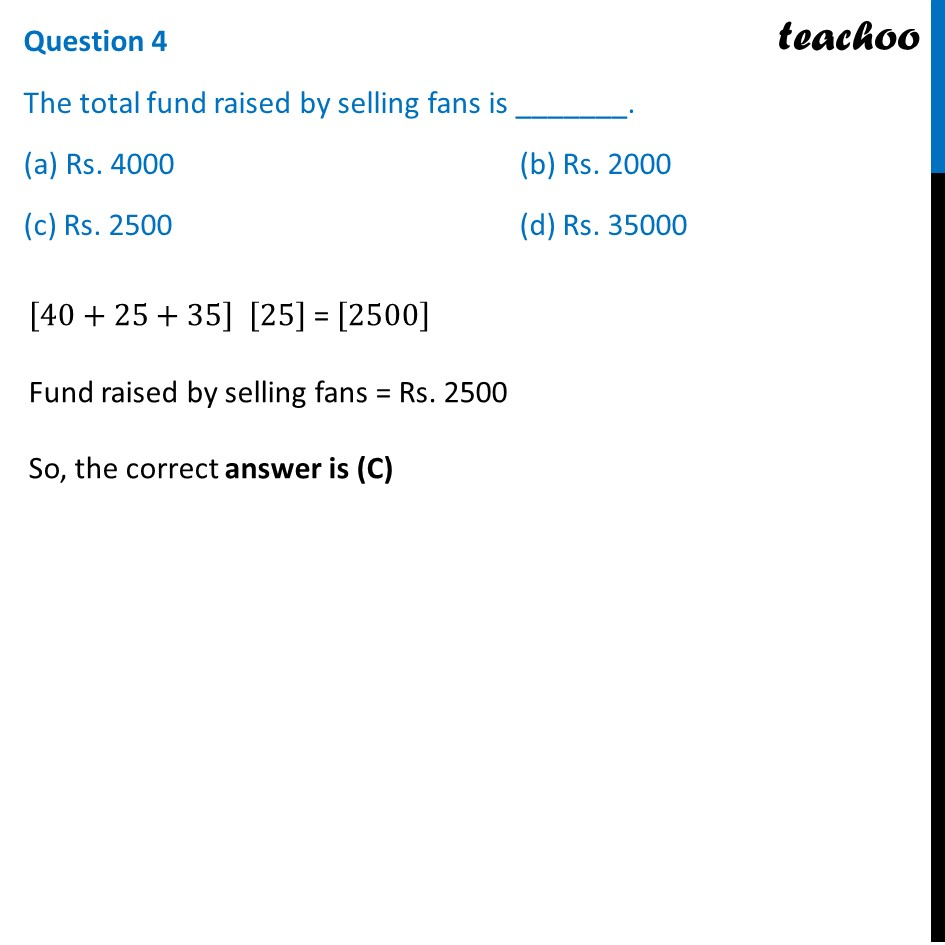## (d) Rs. 35000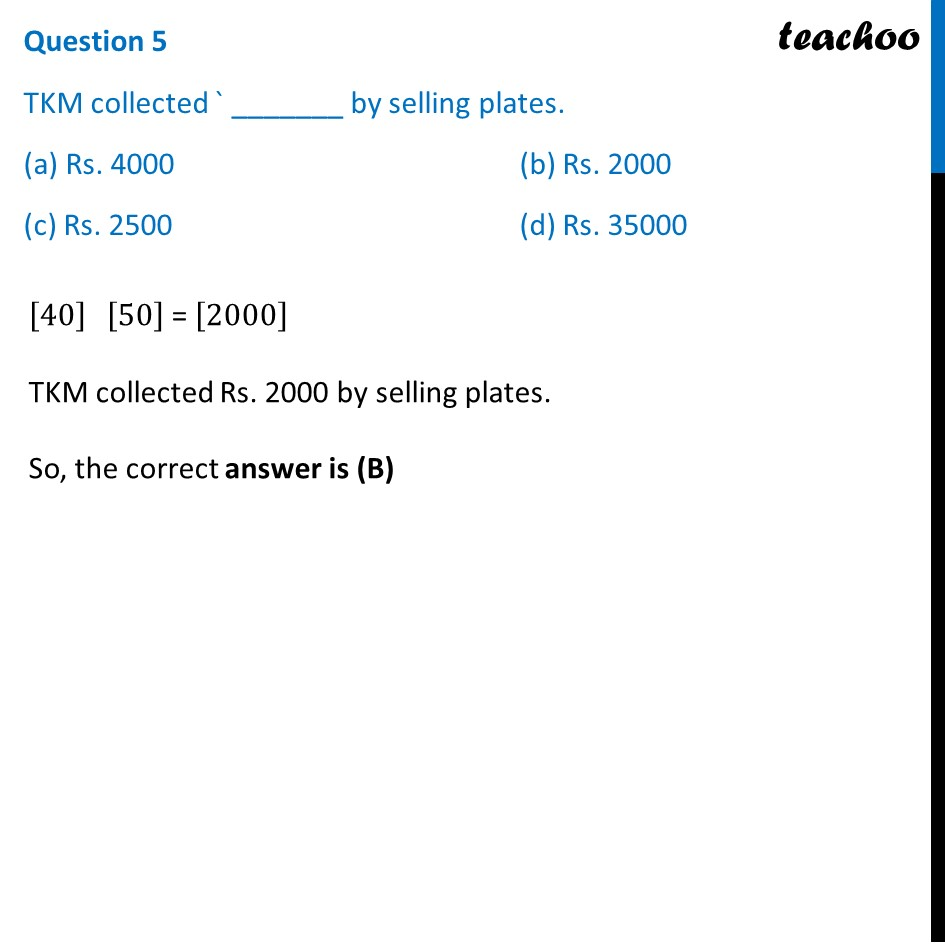Learn in your speed, with individual attention - Teachoo Maths 1-on-1 Class

### Transcript

Question Three schools SNT, SNP and TKM organised a mela for collecting funds for helping the rehabilitation of flood victims. They sold hand-made fans, mats and plates from recycled material at a cost of ₹ 25, ₹ 100 and ₹ 50 each. The number of articles sold are given below. Question 1 Funds collected by SNT is _______. (a) Rs. 7000 (b) Rs. 6125 (c) Rs. 7875 (d) Rs. 21000 Let the number of articles sold be denoted by matrix X X = [■8(40& 25& 35@50& 40& 50@20& 30& 40)] Let the sale price of articles be denoted by matrix Y Let Y = [■8(25& 100& 50)] Now, Total Revenue = Sales price × Total sales = YX = [■8(25&100&50)]_(1×3) [■8(40& 25& 35@50& 40& 50@20& 30& 40)]_(3×3) = [■8(25(40)+100(50)+50(20)&25(25)+100(40)+50(30)&25(35)+100(50)+50(40))]_(1×3) = [■8(1000+5000+1000&625+4000+1500&875+5000+2000)] = [■8(𝟕𝟎𝟎𝟎&𝟔𝟏𝟐𝟓&𝟓𝟖𝟕𝟓)] ∴ Total amount collected by SNT = Rs 7,000 So, the correct answer is (a) Question 2 Funds collected by SNP is _______. (a) Rs. 7000 (b) Rs. 6125 (c) Rs. 7875 (d) Rs. 21000 Total Revenue = [■8(𝟕𝟎𝟎𝟎&𝟔𝟏𝟐𝟓&𝟓𝟖𝟕𝟓)] Thus, Fund raised by SNP = Rs. 6125 So, the correct answer is (B) Question 3 The total fund raised by all the three schools together is _______. (a) Rs. 7000 (b) Rs. 6125 (c) Rs. 7875 (d) Rs. 21000 Now, Total Revenue = [■8(7000&6125&5875)] Thus, Total Amount collected by all 3 schools = Rs 7000 + 6125 + 5875 = Rs 21,000 So, the correct answer is (d) Question 4 The total fund raised by selling fans is _______. (a) Rs. 4000 (b) Rs. 2000 (c) Rs. 2500 (d) Rs. 35000 Fund raised by selling fans = Total fans × Cost of selling fan = (40 + 25 + 35) × Rs 25 = 100 × 25 = Rs. 2500 So, the correct answer is (C) Question 5 TKM collected Rs _______ by selling plates. (a) Rs. 4000 (b) Rs. 2000 (c) Rs. 2500 (d) Rs. 35000 Fund raised by TKM for selling plates = Total plates sold by TKM × Cost of selling plates = 40 × Rs 50 = Rs. 2000 So, the correct answer is (b)